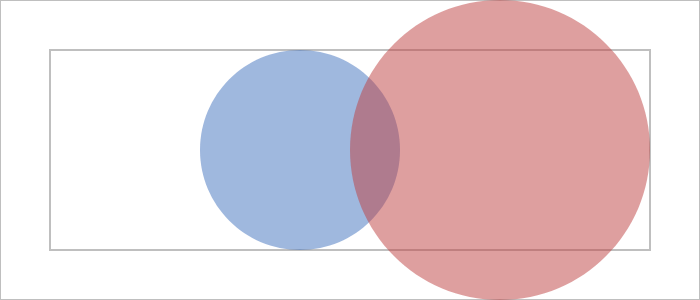# Geometry

## Dividing Rect(angles)

One of the core concepts of Coldtype is the use of the `coldtype.geometry.Rect` class to encapsulate rectangles and methods for slicing & dicing them.

The most basic rectangle is the one passed to a `renderable`, i.e. the `r` variable you get when you define a renderable function, like `def r1(r)` below. So to fill the entire canvas with a single random color, you can do something like this:

```@renderable((700, 300))
def r1(r):
return P(r).f(hsl(random()))
```## Inset, offset, take, divide, subdivide…

All `@renderables` have a rectangle associated with them (the full rectangle of the artifact canvas), and all rendering functions are passed rectangles, either via the first and only argument, or as a property of the first argument, as is the case with @animation renderables, which pass a Frame argument that makes the rectangle accessible via f.a.r (where f is the Frame).

But we’re getting ahead of ourselves.

A Rect has lots of methods, though the most useful ones are `inset`, `offset`, `take`, `divide`, and `subdivide`.

Here’s a simple example that insets, offsets, subtracts, and then subtracts again. (Probably not something I’d write in reality, but good for demonstration purposes.)

```@renderable((700, 300))
def r2(r):
r1 = (r.take(0.5, "W") # "W" for "West"
.inset(20, 20)
.offset(0, 10)
.subtract(20, "E")
.subtract(10, "N"))

return (P().rect(r1)
.f(hsl(0.5)))
```## More complex slicing & dicing

You may have noticed that the rect functions take a mix of float and int arguments. That’s because a value less than `1.0` will be treated, by the dividing-series of rect functions, as percentages of the dimension implied by the edge argument. So in that `take(0.5, "W")` above, the `0.5` specifies 50% of the width of the rectangle (width because of the `W` edge argument).

Here’s an example that divides a rectangle into left and right rectangles, and shows another useful method, `square` (which takes the largest square possible from the center of the given rectangle).

```@renderable((700, 300))
def lr(r):
ri = r.inset(50, 50)
left, right = ri.divide(0.5, "W")
return P(
P().rect(ri).fssw(-1, 0.75, 2),
P().oval(left
.square()
.offset(100, 0))
.f(hsl(0.6, a=0.5)),
P().oval(right
.square()
.inset(-50))
.f(hsl(0, a=0.5)))
```Here’s an example using subdivide to subdivide a larger rectangle into smaller pieces, essentially columns.

```@renderable((700, 300))
def columns(r):
cs = r.inset(10).subdivide(5, "W")
return P.Enumerate(cs, lambda x:
P(x.el.inset(10)).f(hsl(random())))
```Of course, columns like that aren’t very typographic. Here’s an example using subdivide_with_leading, a useful method for quickly getting standard rows or columns with classic spacing.

```@renderable((700, 500))
return P.Enumerate(cs, lambda x:
P(x.el).f(hsl(random())))
```## Grids

If you’re a fan of CSS grids, you might like the weird little `Grid` class made available in Coldtype.

```r = Rect(700, 300)
g = Grid(r, "a a", "a a", "a b / c d")

@renderable(r)
def simple_grid1(r):
return P(
P(g["a"]).f(hsl(0)),
P(g["d"]).f(hsl(0.5)))
``````g = Grid(r, "a 100", "100 a", "a b / c d")

@renderable(r)
def simple_grid2(r):
return (
P(g["a"]).f(hsl(0)),
P(g["d"]).f(hsl(0.5)))
``````g = Grid(r, "a 100 a", "a 100 a", "a b c / d e f / g h i")

@renderable(r)
def simple_grid3(r):
return (
P(g["a"]).f(hsl(0)),
P(g["e"]).f(hsl(0.3)),
P(g["i"]).f(hsl(0.5)))
```